# It's too late to go into the pit. What's the simplest neural network like?

2 articles 9 subscription

catalogue

1. Neural network training process

2. Basic concepts

3. Data preprocessing means

4. Data processing library

5. Training set, test set, test set

5. Loss function

6. Optimizer

7. Activation function

8、hello world

9. Summary

Recommended reading    Click the title to jump

Today is the first article. I hope I can stick to it. Come on.

Deep neural network uses a set of functions to approximate the original function, and the training process is the process of finding parameters.

### 1. Neural network training process

The training process of neural network is as follows:

• Collect and organize data

• The neural network is used to fit the objective function

• Make a loss function that directly estimates the error between the real value and the objective function value, and generally select the established loss function

• Using the loss function value to derive from the input value,

• Then update the network parameter (x) according to the opposite direction of the derivative, so that the loss function value is finally 0, and finally generate the model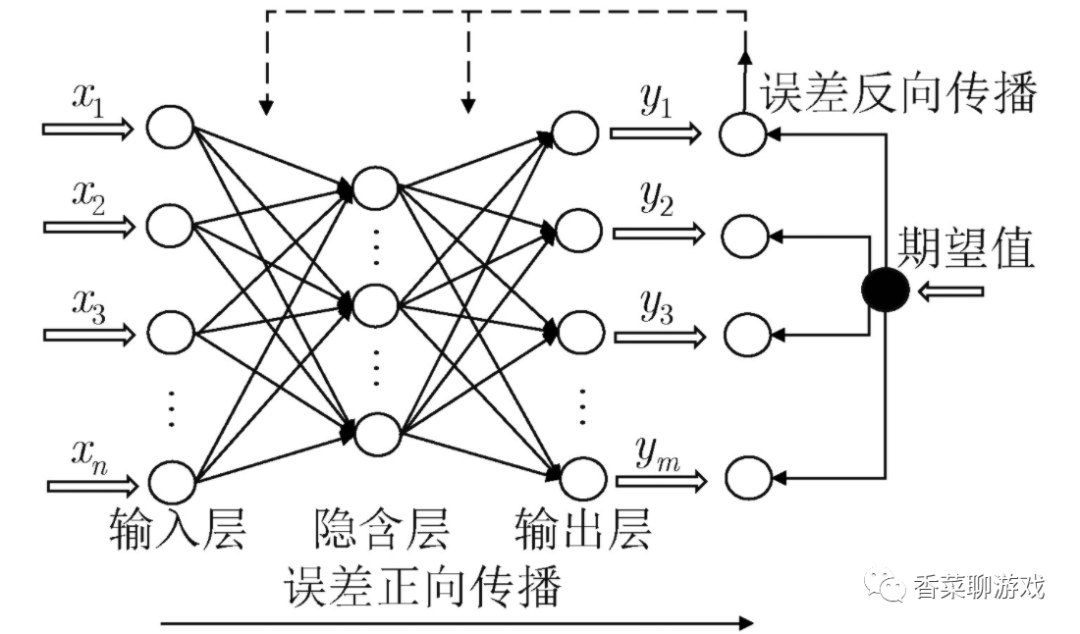Concept explanation of each layer

• Input layer: parameter input

• Output layer: the final output

• Hidden layer (hidden layer): layers other than the other two layers can be called hidden layers

What is the model:

• The model consists of two parts, one is the structure of neural network, the other is each parameter, and the final training result is this

### 2. Basic concepts

1. Mathematical knowledge

1.1 derivative

I learned derivative in college. Although the concept is very simple, I almost forgot it after so many years. I don't even remember the mathematical symbols. I didn't understand it until I reviewed it: refers to the speed of data change, which is the concept of change rateFor example, the acceleration of gravity represents the increment of velocity per second after you fall freely.

The mathematical formula is: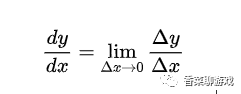It's not important. You can understand it whether you read it or not, because you won't be asked to calculate the derivative manually in the later study. There are ready-made functions in the framework

The original meaning of gradient is a vector (vector), which represents the direction of a function at the point, and the derivative reaches the maximum along the direction, that is, the function changes the fastest along the direction (the direction of the gradient) at the point, and the change rate is the largest (the modulus of the gradient)

Gradient: it is a vector with the largest directional derivative in its direction, and its size is exactly the largest directional derivative.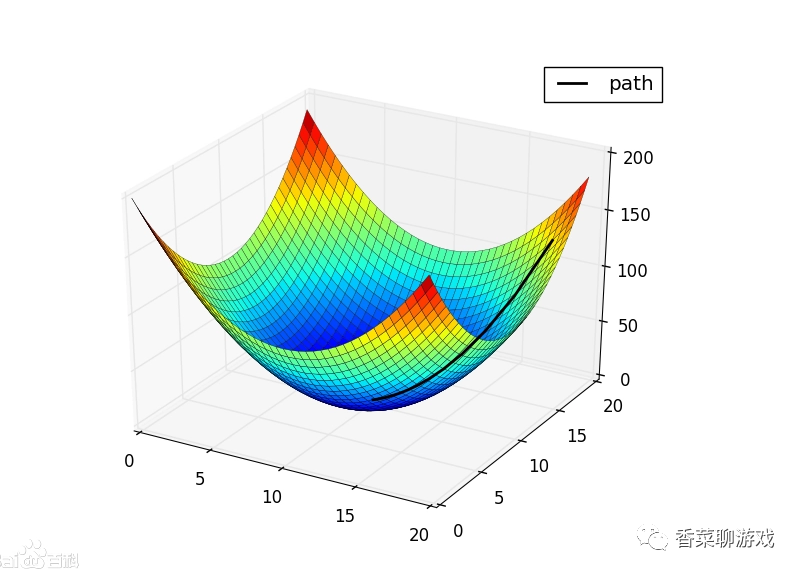2. Forward and backward propagation

Forward propagation is a forward call. It's just a normal function call chain. It's nothing special. It's mysterious to break the concept

such as

def   a(input):
return   y

def   b(input):
return   y2
#Forward propagation
def   forward(input):
y  =  a(input)
y2  =  b(y)

Back propagation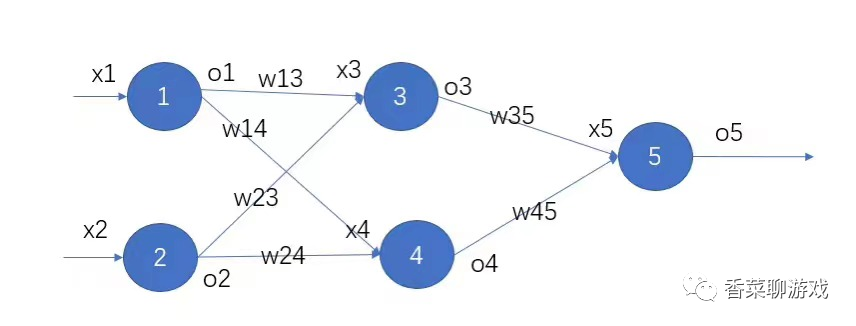Back propagation is to adjust the parameter weight according to the error and learning rate. The specific algorithm will be analyzed in an article next time.

### 3. Data preprocessing means

3.1 normalization

Reduce the data to 0 ~ 1 interval, and use the formula (x-min) / (max min)

3.2 standardization

Data standardization is to scale the data to a small specific interval. The data is transformed into a standard normal distribution with a mean of 0 and a variance of 1

3.3 regularization

The main function of regularization is to prevent over fitting. Adding regularization term to the model can limit the complexity of the model and balance the complexity and performance of the model.

3.4 one hot code

One hot coding is an easy-to-use process of converting category variables into machine learning algorithms.one-hotUsually used for feature conversion

For example, seven days a week, the third day can be coded as [0,0,1,0,0,00]

Note: I made up all my English behind, not to pretend to be forced, but to know what the word means when I see it next time.

### 4. Data processing library

Numpy, pandas and Matplotlib are three libraries commonly used in data analysis and deep learning

4.1 numpy

Numpy is a list of optimized python, which not only improves the running efficiency, but also provides many convenient functions. Generally, it represents the matrix when used

An important concept in numpy is called shape, that is, representing dimension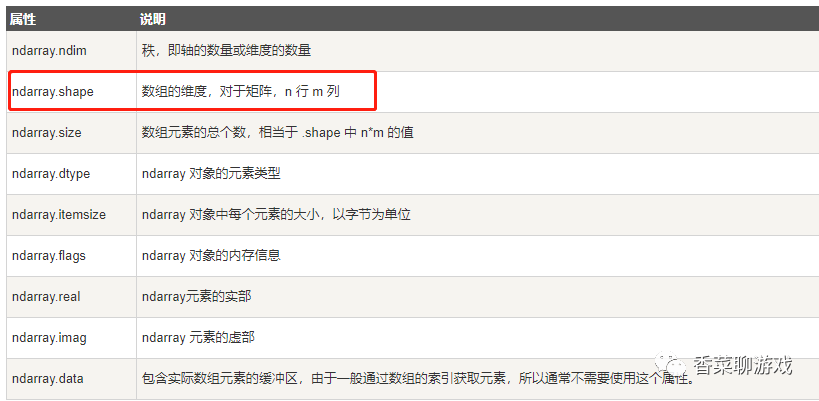Note: I am not proficient in using numpy API. I believe I will be proficient in the future learning process. Check it when using it. Don't worry.

4.2 pandas

The main data structures of pandas are series (one-dimensional data) and dataframe (two-dimensional data)

[Series]Is an object similar to a one-dimensional array. It consists of a set of data (various numpy data types) and a set of associated data labels (i.e. indexes).

DataFrameIs a tabular data structure. It contains a set of ordered columns. Each column can be of different value types (numeric value, string, Boolean value). Dataframe has both row indexes and column indexes. It can be regarded as a dictionary composed of series (using a common index).

Note:Pandas can be used as excel, I'm not proficient in the API. Don't worry. Just sweep down the core concepts

4.3 matplotlib

Matplotlib   It's for drawing pictures and can be used to visualize data in the process of learning. I haven't learned this library yet. I can only follow the cat and draw the tiger, so relax. I just tell you that there is such a thing that you don't have to master it now

### 5. Training set, test set, test set

Training set: the data used to train the model for learning

Validation set: the data used to validate the model, mainly to see the training of the model

Test set: after training, verify the data of the model

The ratio of general data is 6:2:2

A vivid metaphor:

Training set - students' textbooks; Students master knowledge according to the contents of the textbook.

Verification set - homework, through which we can know the learning situation and progress speed of different students.

Test set - test, the test questions are usually not seen, to investigate the students' ability to draw inferences from one instance.

### 5. Loss function

loss function Used to evaluate the modelEstimateandTrue valueDifferent degrees, the better the loss function, the better the performance of the model. The loss functions used in different models are generally different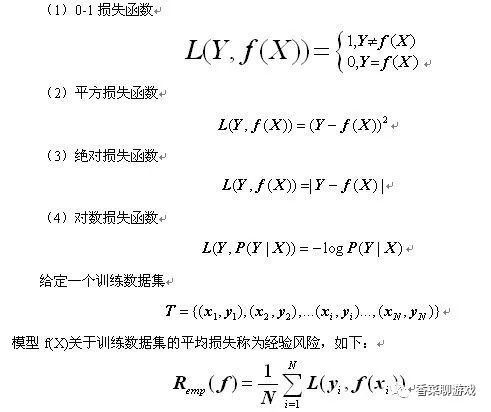Note: F (x) represents the predicted value, Y represents the real value,

These are just common loss functions with different implementations. It's OK to understand each function in the later development. API caller doesn't need to understand the specific implementation, just like you know the algorithm principle of quick sorting, but it's not necessary to implement it yourself. Isn't it fragrant to use the ready-made implementation?

### 6. Optimizer

In the process of deep learning back propagation, the optimizer guides each parameter of the loss function (objective function) to update the appropriate size in the correct direction, so that the updated parameters make the value of the loss function (objective function) approach the global minimum.

Several common optimizers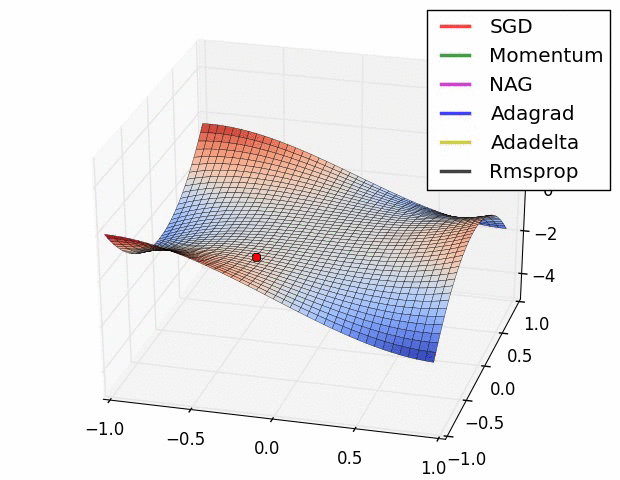### 7. Activation function

The activation function is to filter the input, which can be understood as a filter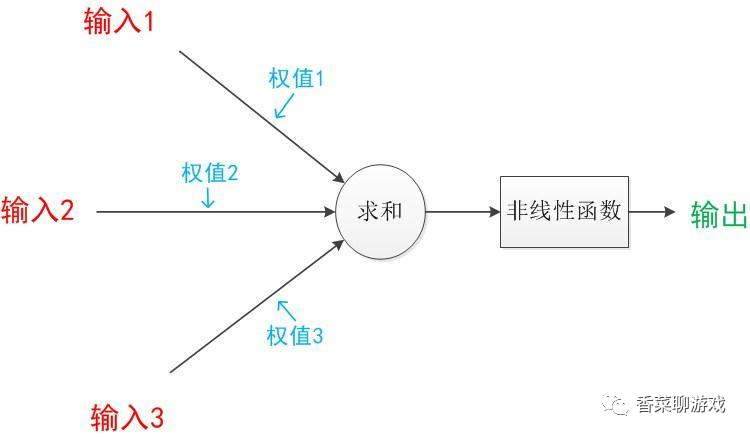Common nonlinear activation functions can be divided into two categories: one is to input a single variable and output a single variable, such as sigmoid function and relu function; The other is to input multiple variables and output multiple variables, such as softmax function and maxout function.

• For the binary classification problem, sigmoid function can be selected in the output layer.

• For multi classification problems, the softmax function can be selected in the output layer.

• Due to the gradient disappearance problem, try to use sigmoid function and tanh.

• Tanh function usually performs better than sigmoid function because it is centered on 0.

• Relu function is a general function, which can be considered in the hidden layer.

• Sometimes it is necessary to modify the existing activation function slightly and consider using the newly discovered activation function.

### 8、hello world

After talking about a lot of concepts, let's have a demo. The following is the simplest linear regression model.

The installation of the environment is at the beginning of the article.

import torch as t
import torch.nn as nn
import torch.optim as optim
import matplotlib.pyplot as plt
import numpy as np

#Learning rate, that is, the size of each parameter movement
lr = 0.01
#Number of training data sets
num_ epochs = 100
#Number of input parameters
in_ size = 1
#Number of output parameters
out_ size = 1
#X dataset
x_ train = np.array([[3.3], [4.4], [5.5], [6.71], [6.93], [4.168],
[9.779], [6.182], [7.59], [2.167], [7.042],
[10.791], [5.313], [7.997], [3.1]], dtype=np.float32)
#True value corresponding to y
y_ train = np.array([[1.7], [2.76], [2.09], [3.19], [1.694], [1.573],
[3.366], [2.596], [2.53], [1.221], [2.827],
[3.465], [1.65], [2.904], [1.3]], dtype=np.float32)
#Linear regression network
class LinerRegression(nn.Module):
def __ init__( self, in_ size, out_ size):
super(LinerRegression, self).__ init__()
self.fc1 = nn.Linear(in_ size, out_ size)

def forward(self, x):
y_ hat = self.fc1(x)
return y_ hat

model = LinerRegression(in_ size, out_ size)
#Loss function
lossFunc = nn.MSELoss()
#Optimizer
optimizer = optim.SGD(model.parameters(), lr=lr)
#Number of cycles to train the dataset
for epoch in range(num_ epochs):
x = t.from_ numpy(x_ train)
y = t.from_ numpy(y_ train)
y_ hat = model(x)
loss = lossFunc(y_ hat, y)
#Derivative zeroing
#Back propagation, that is, modify the parameters and modify the parameters in the correct direction
loss.backward()
optimizer.step()
print("[{}/{}] loss:{:.4f}".format(epoch+1, num_ epochs, loss))

#Draw a picture to see how the final model fits
y_ pred = model(t.from_ numpy(x_ train)).detach().numpy()
plt.plot(x_ train, y_ train, 'ro', label='Original Data')
plt.plot(x_ train, y_ pred, 'b-', label='Fitted Line')
plt.legend()
plt.show()

The above is the simplest linear regression neural network. There is no hidden layer and no activation function.

It runs very fast because there are few parameters. The final results of the operation can be seen. Finally, our results are achieved. You can try to adjust some parameters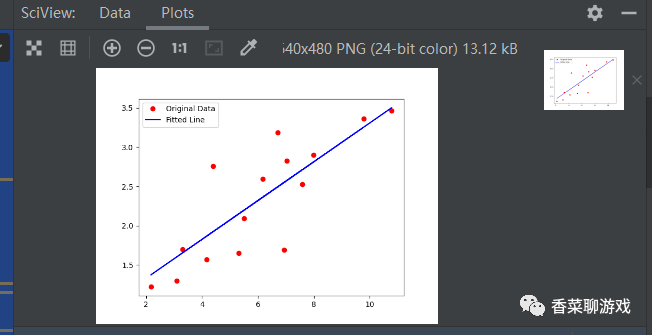### 9. Summary

Today, I wrote a lot of concepts. I don't need to master them all. First, I'll get familiar with them. First, I'll have an overall view. I can understand them slowly. There are many formulas in them. I don't need to understand them. Be easyInsert expression
Can also inputone thousandCharacters
05-30nine hundred and sixty-two
04-21five thousand seven hundred and forty-six
03-15three hundred and forty-six
©️ 2020 CSDN Skin theme: Cool shark Designer: CSDN official blog
Paid inelement
Payment with balanceClick retrieveCode scanning payment
Wallet balance 0Deduction Description:

1. The balance is the virtual currency of wallet recharge, and the payment amount is deducted according to the ratio of 1:1.
2. The balance cannot be purchased and downloaded directly. You can buy VIP, c-coin package, paid column and courses.Balance recharge
sportsinfo4.com